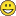# QlikView App Dev

Discussion Board for collaboration related to QlikView App Development.

cancel
Showing results for
Did you mean:Contributor II

## sum of aggr() returns 0

I have an expression which counts difference between beneficiaries for selected year and its previous year.The expression calculates the sum of beneficiaries for this year sum of beneficiaries for previous year and the subtracts both the values to get difference.

The part which calculates sum of beneficiaries for selected year gives correct value.

But the part which calculates beneficiaries for previous year always evaluates to 0.

Part which calculates sum of beneficiaries for selected

sum({<Financial_Year = {'\$(Selected_Year)'}, Quarter = {'\$(Selected_Quarter)'}>} aggr(if(TYPE = 'ABC',count({<Financial_Year = {'\$(Selected_Year)'}, Quarter = {'\$(Selected_Quarter)'}>}distinct BENEFICIARY))

Part which calculates sum of beneficiaries for previous year(which always evaluates to 0)

sum({<Financial_Year = {'\$(Previous_Year)'}, Quarter = {'\$(Selected_Quarter)'}>}aggr(if(TYPE = 'ABC',count({<Financial_Year = {'\$(Previous_Year)'}, Quarter = {'\$(Selected_Quarter)'}>}distinct BENEFICIARY))

I have declared variables as follows

Selected_Year = GetFieldSelections(Financial_Year)

Selected_Quarter = GetFieldSelections(Quarter)

Previous_Year = GetFieldSelections(Financial_Year) - 1

My entire expression is as follows:

=if(GetFieldSelections(Quarter),

sum({<Financial_Year = {'\$(Selected_Year)'}, Quarter = {'\$(Selected_Quarter)'}>}aggr(if(TYPE = 'ABC',count({<Financial_Year = {'\$(Selected_Year)'}, Quarter = {'\$(Selected_Quarter)'}>}distinct BENEFICIARY)) -

sum({<Financial_Year = {'\$(Previous_Year)'}, Quarter = {'\$(Selected_Quarter)'}>}aggr(if(TYPE = 'ABC',count({<Financial_Year = {'\$(Previous_Year)'}, Quarter = {'\$(Selected_Quarter)'}>}distinct BENEFICIARY)),

sum({<Financial_Year = {'\$(Selected_Year)'}>}aggr(if(TYPE = 'ABC',count({<Financial_Year = {'\$(Selected_Year)'}>}distinct BENEFICIARY)) -

sum({<Financial_Year = {'\$(Previous_Year)'}, Quarter = {'\$(Selected_Quarter)'}>}aggr(if(TYPE = 'ABC',count({<Financial_Year = {'\$(Previous_Year)'}>}distinct BENEFICIARY))

Any help will be appreciated1 Solution

Accepted SolutionsMVP

Try this for previous year

Sum({<Financial_Year = {'\$(Previous_Year)'}, Quarter = {'\$(Selected_Quarter)'}>} Aggr(If(Only({1} TYPE) = 'ABC', Count({<Financial_Year = {'\$(Previous_Year)'}, Quarter = {'\$(Selected_Quarter)'}>} DISTINCT  BENEFICIARY))

I think you might be missing the last part of the expression where you give the Aggr's dimension/s

5 RepliesMVP

Try this for previous year

Sum({<Financial_Year = {'\$(Previous_Year)'}, Quarter = {'\$(Selected_Quarter)'}>} Aggr(If(Only({1} TYPE) = 'ABC', Count({<Financial_Year = {'\$(Previous_Year)'}, Quarter = {'\$(Selected_Quarter)'}>} DISTINCT  BENEFICIARY))

I think you might be missing the last part of the expression where you give the Aggr's dimension/sContributor II
Author

Yes I am sorry for that

sum({<Financial_Year = {'\$(Selected_Year)'}, Quarter = {'\$(Selected_Quarter)'}>} aggr(if(TYPE = 'ABC',count({<Financial_Year = {'\$(Selected_Year)'}, Quarter = {'\$(Selected_Quarter)'}>}distinct BENEFICIARY)), REFID))

Added the dimension REFIDMVP

Did you try it with the change I said?

Sum({<Financial_Year = {'\$(Previous_Year)'}, Quarter = {'\$(Selected_Quarter)'}>} Aggr(If(Only({1} TYPE) = 'ABC', Count({<Financial_Year = {'\$(Previous_Year)'}, Quarter = {'\$(Selected_Quarter)'}>} DISTINCT  BENEFICIARY)), REFID))Contributor II
Author

Yeah tried it just now and its working.Can you please explain the effect of adding the part in red to the codeMVP

Although other parts of the expression was ignoring selection in current year, but TYPE was not... which is why the expression was not working. Ignored all selection for TYPE as well using Only({1} ... ) and it worked